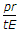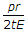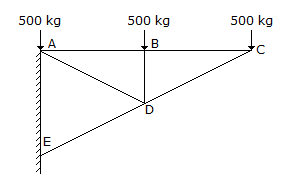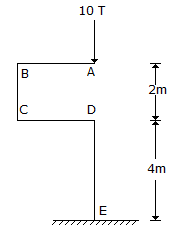# Civil Engineering - Strength of Materials

### Exercise :: Strength of Materials - Section 3

21.

The point of contraflexture occurs in

 A. cantilever beams only B. continuous beams only C. over hanging beams only D. all types of beams E. both (a) and (b).

Explanation:

No answer description available for this question. Let us discuss.

22.

An open-ended cylinder of radius r and thickness t is subjected to internal pressure p. The Young's modulus for the material is E and Poisson's ratio is μ. The longitudinal strain is

 A. zero B.C.D. none of these.

Explanation:

No answer description available for this question. Let us discuss.

23.

The force in BD of the truss shown in below figure is :A. 500 kg compressive B. 500 kg tensile C. 1500 kg tensile D. 1500 kg compressive E. zero.

Explanation:

No answer description available for this question. Let us discuss.

24.

The bending moment at E for the structure shown in below figure, isA. zero B. 10 Tm C. 20 Tm D. 40 Tm.

Explanation:

No answer description available for this question. Let us discuss.

25.

A member which is subjected to reversible tensile or compressive stress may fail at a stress lower than the ultimate stress of the material. This property of metal, is called

 A. plasticity of the metal B. elasticity of the metal C. fatigue of the metal D. workability of the metal.